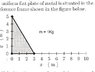# Moment of inertia of a right triangle

• munther

#### munther

in my homework the question wants from me to find the moment of inertia of
right triangle with the y-axis

as shown in the attatchment

note: the mass of the plate is 1 kg

i found the y coordinate of the center of mass of the plate it is 5/3
and i supposed it is the r

so the inertia will be mr^2
=1*(5/3)^2
=25/9
=2.77777 kg m^2

is that right??

#### Attachments

•scan0001.jpg
27.9 KB · Views: 901
If you're calculating the moment of inertia with respect to the y-axis, then it doesn't look right.

Thank you >
but Why??

can you give me a hint??

i found the y coordinate of the center of mass of the plate it is 5/3
and i supposed it is the r

I think it would be better for you to show us how you found that. Also, why do you suppose it is "the r"?

I think it would be better for you to show us how you found that. Also, why do you suppose it is "the r"?

i found it from the first part of the question

i found it from the first part of the question

Now that you found it, please post it.